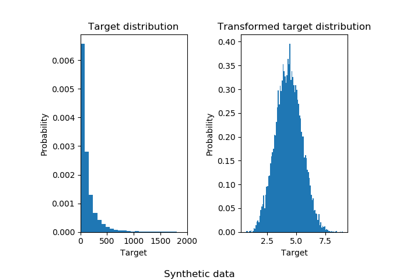# sklearn.compose.TransformedTargetRegressor¶

class sklearn.compose.TransformedTargetRegressor(regressor=None, transformer=None, func=None, inverse_func=None, check_inverse=True)[source]

Meta-estimator to regress on a transformed target.

Useful for applying a non-linear transformation to the target y in regression problems. This transformation can be given as a Transformer such as the QuantileTransformer or as a function and its inverse such as log and exp.

The computation during fit is:

regressor.fit(X, func(y))


or:

regressor.fit(X, transformer.transform(y))


The computation during predict is:

inverse_func(regressor.predict(X))


or:

transformer.inverse_transform(regressor.predict(X))


Read more in the User Guide.

Parameters
regressorobject, default=LinearRegression()

Regressor object such as derived from RegressorMixin. This regressor will automatically be cloned each time prior to fitting.

transformerobject, default=None

Estimator object such as derived from TransformerMixin. Cannot be set at the same time as func and inverse_func. If transformer is None as well as func and inverse_func, the transformer will be an identity transformer. Note that the transformer will be cloned during fitting. Also, the transformer is restricting y to be a numpy array.

funcfunction, optional

Function to apply to y before passing to fit. Cannot be set at the same time as transformer. The function needs to return a 2-dimensional array. If func is None, the function used will be the identity function.

inverse_funcfunction, optional

Function to apply to the prediction of the regressor. Cannot be set at the same time as transformer as well. The function needs to return a 2-dimensional array. The inverse function is used to return predictions to the same space of the original training labels.

check_inversebool, default=True

Whether to check that transform followed by inverse_transform or func followed by inverse_func leads to the original targets.

Attributes
regressor_object

Fitted regressor.

transformer_object

Transformer used in fit and predict.

Notes

Internally, the target y is always converted into a 2-dimensional array to be used by scikit-learn transformers. At the time of prediction, the output will be reshaped to a have the same number of dimensions as y.

Examples

>>> import numpy as np
>>> from sklearn.linear_model import LinearRegression
>>> from sklearn.compose import TransformedTargetRegressor
>>> tt = TransformedTargetRegressor(regressor=LinearRegression(),
...                                 func=np.log, inverse_func=np.exp)
>>> X = np.arange(4).reshape(-1, 1)
>>> y = np.exp(2 * X).ravel()
>>> tt.fit(X, y)
TransformedTargetRegressor(...)
>>> tt.score(X, y)
1.0
>>> tt.regressor_.coef_
array([2.])


Methods

 fit(self, X, y, \*\*fit_params) Fit the model according to the given training data. get_params(self[, deep]) Get parameters for this estimator. predict(self, X) Predict using the base regressor, applying inverse. score(self, X, y[, sample_weight]) Return the coefficient of determination R^2 of the prediction. set_params(self, \*\*params) Set the parameters of this estimator.
__init__(self, regressor=None, transformer=None, func=None, inverse_func=None, check_inverse=True)[source]

Initialize self. See help(type(self)) for accurate signature.

fit(self, X, y, **fit_params)[source]

Fit the model according to the given training data.

Parameters
X{array-like, sparse matrix}, shape (n_samples, n_features)

Training vector, where n_samples is the number of samples and n_features is the number of features.

yarray-like, shape (n_samples,)

Target values.

**fit_paramsdict of string -> object

Parameters passed to the fit method of the underlying regressor.

Returns
selfobject
get_params(self, deep=True)[source]

Get parameters for this estimator.

Parameters
deepbool, default=True

If True, will return the parameters for this estimator and contained subobjects that are estimators.

Returns
paramsmapping of string to any

Parameter names mapped to their values.

predict(self, X)[source]

Predict using the base regressor, applying inverse.

The regressor is used to predict and the inverse_func or inverse_transform is applied before returning the prediction.

Parameters
X{array-like, sparse matrix} of shape (n_samples, n_features)

Samples.

Returns
y_hatarray, shape = (n_samples,)

Predicted values.

score(self, X, y, sample_weight=None)[source]

Return the coefficient of determination R^2 of the prediction.

The coefficient R^2 is defined as (1 - u/v), where u is the residual sum of squares ((y_true - y_pred) ** 2).sum() and v is the total sum of squares ((y_true - y_true.mean()) ** 2).sum(). The best possible score is 1.0 and it can be negative (because the model can be arbitrarily worse). A constant model that always predicts the expected value of y, disregarding the input features, would get a R^2 score of 0.0.

Parameters
Xarray-like of shape (n_samples, n_features)

Test samples. For some estimators this may be a precomputed kernel matrix or a list of generic objects instead, shape = (n_samples, n_samples_fitted), where n_samples_fitted is the number of samples used in the fitting for the estimator.

yarray-like of shape (n_samples,) or (n_samples, n_outputs)

True values for X.

sample_weightarray-like of shape (n_samples,), default=None

Sample weights.

Returns
scorefloat

R^2 of self.predict(X) wrt. y.

Notes

The R2 score used when calling score on a regressor will use multioutput='uniform_average' from version 0.23 to keep consistent with r2_score. This will influence the score method of all the multioutput regressors (except for MultiOutputRegressor). To specify the default value manually and avoid the warning, please either call r2_score directly or make a custom scorer with make_scorer (the built-in scorer 'r2' uses multioutput='uniform_average').

set_params(self, **params)[source]

Set the parameters of this estimator.

The method works on simple estimators as well as on nested objects (such as pipelines). The latter have parameters of the form <component>__<parameter> so that it’s possible to update each component of a nested object.

Parameters
**paramsdict

Estimator parameters.

Returns
selfobject

Estimator instance.

## Examples using sklearn.compose.TransformedTargetRegressor¶